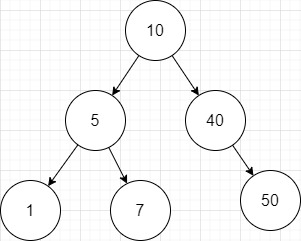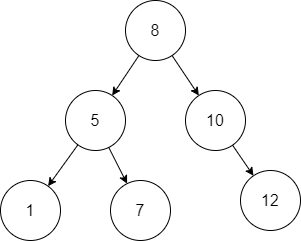Problem title
Difficulty
Avg time to solve

Sum of Two Elements Equals the Third.
Easy
10 mins
Number of balanced binary trees
Easy
10 mins
Search a 2D Matrix II
Easy
15 mins
Goku and Dragon Balls
Moderate
35 mins
Word Break
Moderate
15 mins
Remove Duplicates from Sorted Array
Easy
15 mins
Sub Matrices With Sum Zero
Moderate
15 mins
Combination Sum II
Moderate
30 mins
Minimum Swaps
Moderate
10 mins
Shuffle Two Strings
Hard
50 mins11

# Construct BST from Preorder Traversal

Difficulty: MEDIUM

Problem Statement

#### You are given a preorder traversal of a binary search tree, your task is to find the tree from the given preorder traversal.

##### For example:
``````You are given preOrder = [10, 5, 1, 7, 40, 50], the binary search tree from the given preorder traversal is
``````##### Input Format:
``````The first line of input contains a single integer ‘T’ representing the number of test cases.

The first line of each test case contains a single integer ‘N’ representing the size of the preorder array.

The second line of each test case contains ‘N’ space-separated integers representing the preorder traversal of the tree.
``````
##### Output Format:
``````For each test, ‘N’ space-separated integers will be printed representing the inorder traversal of the tree.

Print a separate line for each test case.
``````
##### Constraints:
`````` 1 <= T <= 10
1 <= N <= 10^6
1 <= preOrder[i] <= 10^9

It is guaranteed that the sum of ‘N’ over all test cases does not exceed 10^6.

Time Limit: 1 sec.
``````
##### Note:
``````You do not need to print anything. It has already been taken care of. Just implement the function.
``````
##### Sample Input 1
``````2
6
10 5 1 7 40 50
6
8 5 1 7 10 12
``````
##### Sample Output 1:
``````1 5 7 10 40 50
1 5 7 8 10 12
``````
##### Explanation:
``````For the first test case,  preOrder = [10, 5, 1, 7, 40, 50], the binary search tree from the given preorder traversal is
````````````Hence the answer is [1, 5, 7, 10, 40, 50].

For the second test case,  preOrder = [8, 5, 1, 7, 10, 12], the binary search tree from the given preorder traversal is
````````````Hence the answer is [1, 5, 7, 10, 40, 50].
``````
##### Sample Input 2:
``````2
3
2 1 3
3
1 3 2
``````
##### Sample Output 2:
``````1 2 3
1 2 3
``````Console